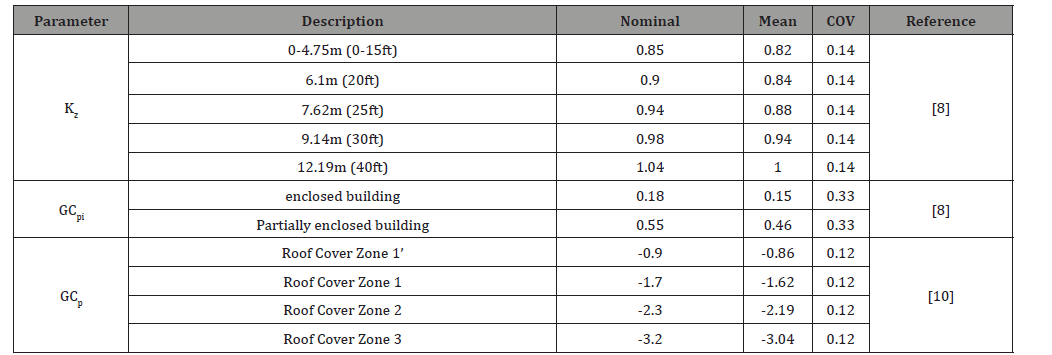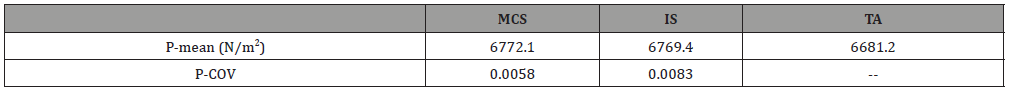# Stochastic Modeling of Uncertainties in the Wind Load Pressure on Residential Buildings Using Different Stochastic Techniques

Omar M Nofal*

Received Date: August 21, 2020;  Published Date: September 10, 2020

#### Abstract

There are different methods to quantify the amount of uncertainties in the analysis of structures and their design procedures. The efficiency and the suitability of each method depend on the type of analysis and the load scenario that will be used for this analysis. In this paper, different uncertainty propagation methods are used to quantify the amount of uncertainties in the wind load analysis procedures to quantify the load impact on the gable roofs of residential buildings. Therefore, Direct Monte Carlo Simulation, Importance Sampling, First Order Reliability Method, and Taylor Approximation are used to further investigate the impact of each method on the uncertainty quantification. The selected example structure to perform the analysis is one of the different archetypes that has been used for the tornado analysis in the literature. The different wind load demand parameters and components statistics are used to conduct the different stochastic analyses. The analysis results showed that MCS is the most efficient method with the least coefficient of variation in the simulated wind pressure and the calculated failure probabilities associated with each stochastic model.

Keywords: Wind load analysis; Direct monte carlo simulation (MCS); Markov chain monte carlo (MCMC); First order reliability method (FORM); Subset analysis; Importance sampling

#### Introduction#### Problem Characterization

There are wide different stochastic simulation techniques that could be used to quantify the amount of uncertainties within engineering systems. However, the main challenge is choosing the most suitable and efficient technique that fit each specific system and the characteristics of its parameters with the lowest number of simulations. Therefore, different stochastic models should be tested, and their analysis results should be compared to determine the most accurate and suitable techniques. In terms of wind load, when buildings are designed for wind, the wind pressure is the main characteristic load that buildings’ components get designed for. The most challenging aspect of the wind pressure quantification is the diversity of parameters included within the pressure equation that accounts for different types of buildings and wind load characteristics. Based on the ASCE7-16 , the extreme wind loads are calculated using Eqs. (1) and (2). The wind load analysis conducted herein does not include the tornado factors that have been included in multiple researches related wind hazards induced by tornados [13,25] as shown in Eq. (3). Therefore, the tornado factors are assumed to be 1.0 to make the analysis more general and simple.where qh = the wind velocity pressure calculated at mean roof height h; Kh= velocity pressure exposure coefficient atmean roof height; Kzt = topographic factor which is assumed to be deterministic and equal to 1.0 in this study; Kd = directionality factor which is also assumed to be deterministic and equal to 0.85; V = 3-s gust wind speed; G = gust-effectfactor;GCp= external pressure coefficient; GCpi= internal pressure coefficient. In the model presented herein, the uncertainty within Kh,GCp, and GCpi were propagated using the statistical models provided in these references[8, 10, 24] as shown in (Table 1).

Wind loads mainly impact the building envelop including walls sheathing and framing, roof sheathing and framing, doors, and windows. The behavior of these components controls the damage state (DS) of buildings subjected to wind loads as described herein [9, 13, 22, 23]. However, the most vulnerable building component is the exterior components. Therefore, the focus of this study is spotted on the roof sheathing, plywood covering, doors, and windows. A wood-frame residential building archetype developed by Amini and van de Lindt  is used herein as an example to illustrate the analysis approach. Table 2 lists the statistical models for some of these components which are provided by Unnikrishnan and Barbato  and Lee and Rosowsky . Afterwards, different stochastic methods were used to account for the different uncertainties within the wind load parameters such as MCS, IS, FORM, and TA.Table 2:Components resistance statistics.#### Structure Definition and Numerical Models

The archetype selected herein is this study is one of the most common one-story residential building adopted in the US buildings industry. It is a one-story single-family wood residential building with a small rectangular plan of (15.3m × 6.9m) with a total area of 105 m2 (1130 ft2). The readers are referred to these articles [8, 22] for more details about the statistical parameters for the resistance of this archetype. Fig. 1 shows detailed dimensions of the chosen archetype. Additionally, the wind load parameters statistics are presented in Table 1 and the building components resistance statistics are presented in (Table 2).

#### Methodology

First, the wind load pressure parameters statistics and the component’s resistance statistics were collected from the literature as discussed above. The mean value and the coefficient of variation of the wind pressure were calculated using MCS, IS, FORM, and TA with the goal of investigating the impact of the different stochastic modeling techniques on the resulted variation in the value of the wind pressure. The mean value was calculated by evaluating the general stochastic integral in Eq. 4 Then, the failure probability of the roof sheathing was calculated and compared the results from adopted methods.where f(ξ) = the probability density function of the estimated parameter, g(ξ) = the scalar function of the random variable, and Ef[g(ξ)] of the random variable.

#### Direct Mont Carlo Simulation analysis

The Direct Monte Carlo simulation (MCS) technique depends on the Central Limit Theorem which includes developing a number of simulations for each random variable to quantify the estimator for Eq. (3). MCS is used to calculate the average value of the integral (Hk) by dividing the total number of the simulated values of the scalar function of the random variable (ξ) by the total number of simulations using Eq. (5). The MCS process conducted herein included generating 1000 samples in a standard Gaussian space to evaluate the wind pressure at each sample point. Then, the mean value and the coefficient of variation for the wind pressure was calculated. The coefficient of variation is calculated using Eq.(6).#### Importance Sampling Analysis

The Importance Sampling (IS) technique is used to generate samples that are within the important region which will help to reduce the variance induced by MCS. Therefore, usually, the COV associated with IS estimator is lower than the one calculated using MCS. Using IS will help to find an optimal distribution that matches the original one by assuming a distribution close enough to the peak of the integrand. The optimal sampling density is assumed to have a positive relationship with the integrand in Eq.(4) whichis calculated using Eq.(7).#### Taylor Approximation

Taylor Approximation estimates the stochastic integral in Eq. (4) using Taylor series. The main goal of using Taylor series is to make the stochastic integral easier and suitable for the differentiation process by including exponential in the integrand as shown in Eq.(8). Then, the estimator for Eq. (4) was evaluated in Eq. (9) by using the second-order Taylor Polynomial and optimizing the integrand to calculate the Hessian Hlnk(ξ)where k(ξ*) = is the value of the integrand function at ξ*, and Hlnk(ξ*) is the Hessian of the logarithmic value of the integrand evaluated at ξ*.

#### First Order Reliability Method

The First Order Reliability Method (FORM) depends on the simplification of the integrand using the idea that the probability density function gets decreased as we move away from the center of the distribution. In this method, a failure region F is assumed to be bounded by a certain threshold and there are some points ξ located within this threshold and other points ξ* with highest probability density function value. Hence, the points around ξ* will exhibit more contribution to the failure probability. The failure probability is the approximation of the F calculated from Eq.(10) which is a function of the reliability index 𝛽 calculated from Eq.(11). {#### Failure Probability Calculation

In the last part, different stochastic modeling methods were investigated to calculate the mean value of the wind pressure at the roof of the impacted residential buildings. The roof failure analysis is conducted by assuming the wind velocity to be 70.0 m/s which represents a category 4 hurricane on the Saffir-Simpson hurricane wind scale or category 3 tornado on the enhanced Fujita scale. Then, the calculated wind pressure will be compared with the resistance of the roof sheathing to calculate the failure probability of this component. The failure probability was calculated using failure indicator function F (ξ). The indicator function is equal to 1.0 in case of failure at wind pressure exceeding the resistance pressure value and is equal to 0.0 in case of no failure at wind pressure less than resistance pressure value. The failure probability will be calculated using Eq. (12).The methods used to evaluate the mean value of the wind pressure were used to calculate the failure probability by replacing g(ξ) with IF (ξ). In addition to these methods, the first-order reliability method was also used to calculate the failure probability.

#### Results

An algorithm that formulates these stochastic models was created using the static models of both wind demand parameters and the roof sheathing statistics. The number of simulations used to conduct the analyses related to each stochastic model is 1000 simulation. The mean value and COV of the wind pressure were calculated using MCS, IS, and Taylor Approximation . (Table 3) Summarizes the wind pressure results calculated from the different stochastic methods. The analysis results show that all the used methods gave very close results. However, MCS gave less coefficient of variation.

Table 3:The resulted mean wind pressure value and C.O.V using MCS, IS, and Taylor Approximation for the roof sheathing.Figure 2 shows how the increase in the number of samples will result in stabilizing the value of the mean wind pressure. Increasing the number of samples is also increasing the efficiency of both MCS and IS method in reducing the coefficient of variation with the adopted number of samples. To that end, MCS is considered the most efficient with less COV. The failure probability for the different components of the selected residential building archetype was then tested using the same methods that have been used before in addition to the FORM method (Table 4). Presents the summary of the results from the different stochastic methods for the select building components. The results showed that the MCS is as efficient as the IS approach which gives almost the same variation resulted from the IS.

Table 4:The failure probability of the different building components along with the C.O.V using MCS, IS, and FORM.#### Summary and conclusion

The overarching goal of this study is to propagate uncertainties within the wind load pressure parameters using different stochastic simulation techniques to capture the impact of using different techniques on the wind pressure mean value and its variation. Therefore, Direct Monte Carlo Simulation, Importance Sampling, and Taylor Approximation were used to pick the efficient technique that will induce less coefficient of variation. Additionally, the failure probability of the building components was further investigated using Direct Monte Carlo Simulation, Importance Sampling, and First Order Reliability Method. The results showed that both Direct Monte Carlo Simulation and Importance Sampling method result in almost the same results in terms of the coefficient of variance of the wind load pressure. However, the MCS method gives less variation with less effort based on the low COV. In terms of failure probability of the different building components, the MCS method showed better efficiency than the other methods based on the resulted low coefficient of variance. So, Monte Carlo simulation did an outstanding performance in the wind pressure calculation with respect to the other methods. That is why all the wind load-based fragility analysis literature is based on the MCS analysis.

None.

#### Conflicts of Interest

No conflict of interest.

Article Details
Citation
Keywords
Scroll to
Scroll to Top